ChemTalk

# Buffer Capacity & Calculations## Core Concepts

In this tutorial, you will learn what a buffer capacity is, and how to calculate it. We will also provide you with a walkthrough of an example problem.

## What is Buffer Capacity?

Buffers are resistant to changes in pH, but they aren’t invincible! Add enough acids or bases into a buffer and its pH will change dramatically.

To understand why this happens, recall that a buffer is a weak acid and its conjugated base (or a weak base and its conjugated acid). Let’s take a look at an example of one such buffer: acetic acid and its conjugated base, acetate.

CH3COOH(aq) ⇄ CH3COO(aq) + H+(aq)

Here, when we add an acid to the buffer, the acetate will absorb the added protons (H+) and form acetic acid, preventing the H+ concentration of the solution and consequentially its pH from changing too much. Likewise, when we add a base to the buffer, the acetic acid will react with the base to form acetate and water, preventing the increase of the OH concentration.

However, these acetic acids and acetates are finite resources. When we add in a whole lot of acid or base, they will use up all of the acetic acid and acetate of the buffer, thus making the buffer susceptible to dramatic pH change.

Buffer capacity, then, is the amount of acid or base we can add to the buffer before its pH dramatically changes. The higher the capacity, the more acid and base we can add to it before its pH changes significantly.The whole point of titration is to overwhelm the buffer capacity of a buffer! Source: Wikimedia Commons.

## General Trends in Buffer Capacity

The higher the concentration of the weak acid and the conjugate base (or a weak base and a conjugate acid) a buffer has, the higher its capacity will be. For example, a buffer with [weak acid] = 0.50 M (the [ ] denotes concentration) and [conjugate base] = 0.30 M would have a higher capacity than a buffer with [weak acid] = 0.050 M and [conjugate base] = 0.030 M.

If a buffer has a greater concentration of the weak acid than that of the conjugate base, then it will have a higher capacity for added base.

Likewise, if a buffer has a greater concentration of the conjugate base than that of the weak acid, then it will have a higher tolerance for added acid. Blood, with its 20:1 ratio of bicarbonate to carbonic acid, is a great example of this type of buffer.

## How to Calculate Buffer Capacity?

In more rigorous terms, buffer capacity is defined as the number of moles of an acid or base that has to be added to 1 liter of a buffer to cause its pH to change by 1 unit.

Therefore, to calculate buffer capacity, we use the following formula: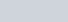• β is buffer capacity (it is unitless)
• n is the number of moles of an acid or base (that were added to the buffer) per liter of the buffer
• ΔpH is the difference between the initial pH of the buffer and the pH of the buffer after the acid or base is added

## Example Problem of Buffer Capacity

Problem:

We are given 600 mL of a sodium phosphate buffer with a pH of 7.39. We then add to it 150 mL of 0.2 M HCl, which gives the buffer solution a new pH of 7.03. What is the capacity of our sodium phosphate buffer?

Solution:

First, we find n by dividing the number of moles of HCl we added to the buffer by the initial volume of the buffer (in liter, don’t forget!).

Number of moles of HCl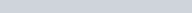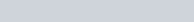Then, following the formula, we divide n by the change in pH of the sodium phosphate solution.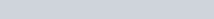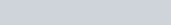Thus, the buffer capacity of our sodium phosphate solution is 0.14.

## Practice Problems

• What is the buffer capacity of a solution that changes 0.5pH units when 0.5 moles of acid are added to 2 liters of the solution.
• What volume of 5M monoprotic acid must be added to change the pH of a buffer solution (strength = 0.22) from 6.7 to 6.3.
• How much buffer solution (strength 0.25) would change pH by 0.73 units when I add 100ml of 3.2M H2SO4.
• CHALLENGE: Using our article on ICE tables, calculate the pH of a buffer solution (initial pH=7, strength = 0.1, volume = 1L), when 100ml of 1M Phosphoric Acid (H3PO4) is added (pKa1=2.15, pKa2=7.20, pKa3=negligible).